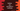# Python set and built in methods : Python tutorial : Part 10## Python Set:

Python Set Introduction : Introduced in python 2.4, Python set is a unordered collection of unique immutable elements. The elements of a set can be anything: numbers, characters, alphabet, other sets etc. A set can be changed. We can add or remove items. We can also perform set operations like differences, intersections etc. between sets.

Creating a Set:

We can create a Set using the built-in set() function or using curly braces. curly braces were introduced in python 2.6.

Using set() function :

``````set1 = set([1,2,3,4,5])
print set1 # set([1, 2, 3, 4, 5])

set2 = set((1,2,3,4,5,4,3,2,1))
print set2 # set([1, 2, 3, 4, 5])

set3 = set("codevscolor")
print set3 # set(['c', 'e', 'd', 'l', 'o', 's', 'r', 'v’])

set4 = set(("one","two","three"))
print set4 # set(['three', 'two', 'one'])``````

In the first example, we have passed a list of numbers to the set() function. In the second example, we have passed a tuple with repeating numbers. But you can see that the same numbers are removed from the set. In the third one, a string is passed. We can also pass specific elements to a python set, as shown in the last example.

All these elements we have used in the above examples are immutable. If you try to use a mutable element as a child of a set, it will throw an error.

Let’s try with curly braces to create a set :

``````set1 = {1,2,3,4,5}
print set1 # set([1, 2, 3, 4, 5])

set2 = {1,2,3,4,5,4,3,2,1}
print set2 # set([1, 2, 3, 4, 5])

set3 = {"codevscolor"}
print set3 # set(['codevscolor'])

set4 = {"one","two","three"}
print set4 # set(['three', 'two', 'one'])``````

Creating an empty set :

We have seen two different ways to create set in python. So, how to create an empty set ? We can use {} and set() , both . But which one to use ? Let’s check :

``````set1 = {}
print type(set1) # <type 'dict'>

set2 = set()
print type(set2) # <type 'set'>
``````

That means, only way to create an empty set is to use the set() method. {} creates an empty dictionary.

Frozensets :

Sets cannot contain any mutable elements, but sets are mutable. Frozensets are similar to sets but they are immutable, i.e. you cannot change a frozenset.

``````set0 = set((1,2,3,4,5))
print set0 # set([1, 2, 3, 4, 5])

print set0 # set([1, 2, 3, 4, 5, 'element'])

set1 = frozenset((1,2,3,4,5))
print set1 # frozenset([1, 2, 3, 4, 5])

Adding elements to a python set :

We can add a single element using add() method , or multiple elements using update() method. Let’s check :

``````set1 = {1,2,3,4}
print set1 # set([1, 2, 3, 4])

print set1 # set([1, 2, 3, 4, 5])

set1.update({"and"},[6,7,8],{9,10,11})
print set1 # set(['and', 1, 2, 3, 4, 5, 6, 7, 8, 9, 10, 11])``````

So, you can see that the update methods can take more than one argument. The argument can be a tuple, list, string or an another python set.

Removing elements from a python set:

We can use discard(element) or remove(element) to remote any set member. In the case of discard, if “element” is not a member of a set, nothing will be done. But, in case of remove(element), it will throw a KeyError.

``````set1 = {1,2,3,4}
print set1 # set([1, 2, 3, 4])

print set1 # set([1, 2, 4])

set1.remove(4)
print set1 # set([1, 2])

set1.remove(4) # KeyError``````

We have two more methods for removing elements in a python set : pop() and clear().

pop() will remove a random element and return it. clear() will remove all elements of a set.

``````set1 = {1,2,3,4,5,6}
print set1 # set([1, 2, 3, 4, 5, 6])

el = set1.pop()
print el # 1

print set1 # set([1, 2, 3, 4, 5, 6])

el2 = set1.pop()
print el2# 2``````

You can see that both times a different element is popped out for the same set.

``````set1 = {1,2,3,4,5}

print set1 # set([1, 2, 3, 4, 5])

set1.clear()

print set1 # set([])``````

Python set union and intersection():

Union of two set is the set of all elements from both sets. An intersection of two set is the set of all common elements between these two sets.

for union , we use union() or ‘|’ operator. For intersection, we use intersection() or ‘&’ operator.

``````set1 = {1, 2, 3, 4}
set2 = {5, 4, 3, 2}

print set1.union(set2) # set([1, 2, 3, 4, 5])
print ( set1 | set2 ) # set([1, 2, 3, 4, 5])

print set1.intersection(set2 # set([2, 3, 4])
print (set1 & set2) # set([2, 3, 4])``````

Set difference and symmetric difference:

For two sets set1 and set2, difference is the elements that are only in set1 but not in set2. Symmetric difference between two sets set1 and set2 is the set of elements of both set1 and set2 except common elements.

To find the difference between two sets, we use “difference()” or “-“ operator. For symmetric difference, we use “symmetric_difference” or “^” operator.

``````set1 = {1, 2, 3, 4}
set2 = {5, 4, 3, 2}

print set1.difference(set2) # set()
print set1 - set2 # set()

print set1.symmetric_difference(set2) # set([1, 5])
print set1 ^ set2 # set([1, 5])``````

Subset and superset check:

set1.issubset(set2) returns True , if set1 is a subset of set2. Similarly, set1.issuperset(set2) returns True if set1 is a superset of set2. We can also use “<=“ for “Subset of” and “>=“ for superset of .

``````set1 = {1, 2, 3}
set2 = {1, 2, 3, 4, 5}

print set1.issubset(set2)
print (set1 <= set2)

print set2.issuperset(set1)
print (set2 >= set1)``````

All print statements will return “True”.